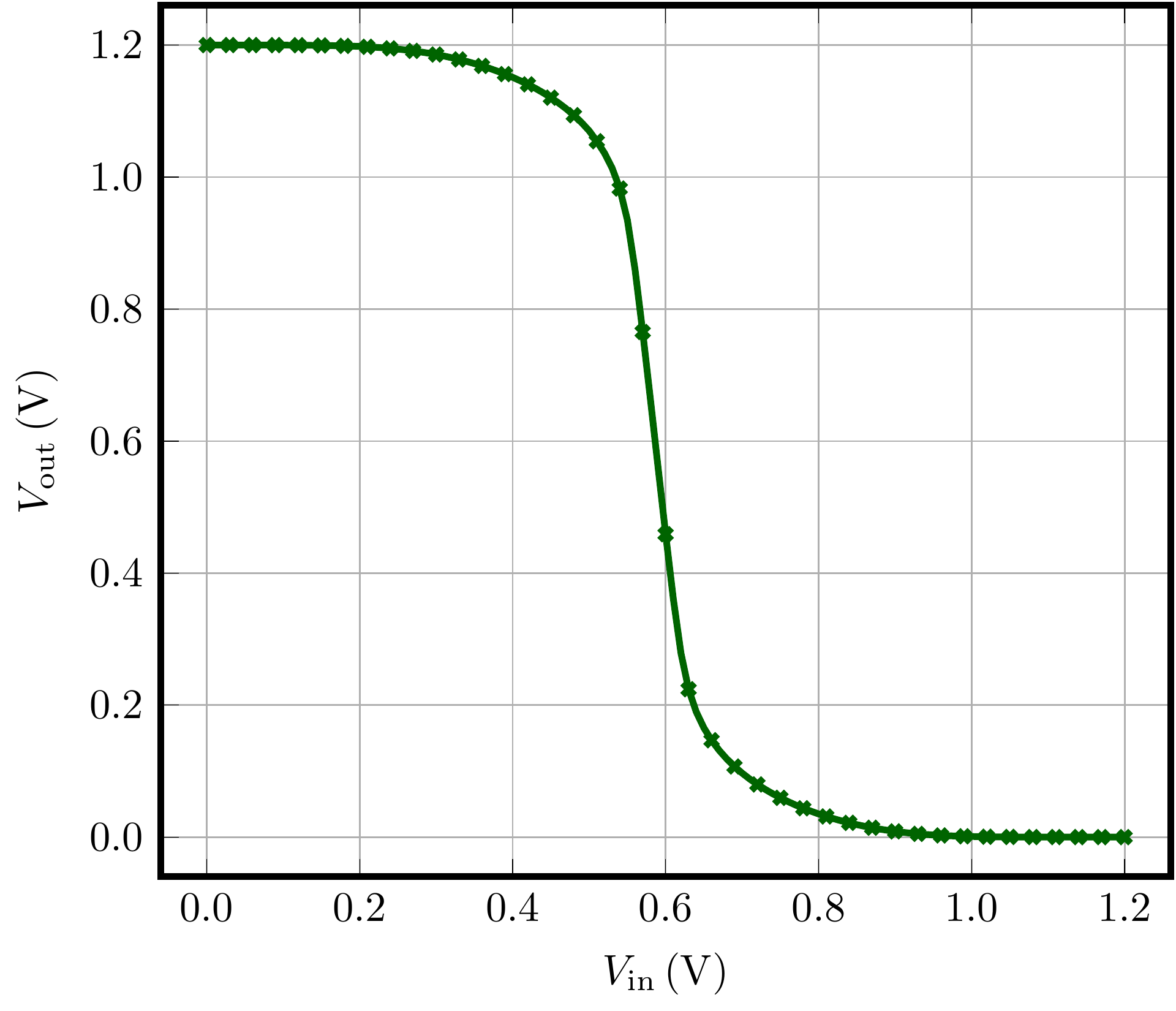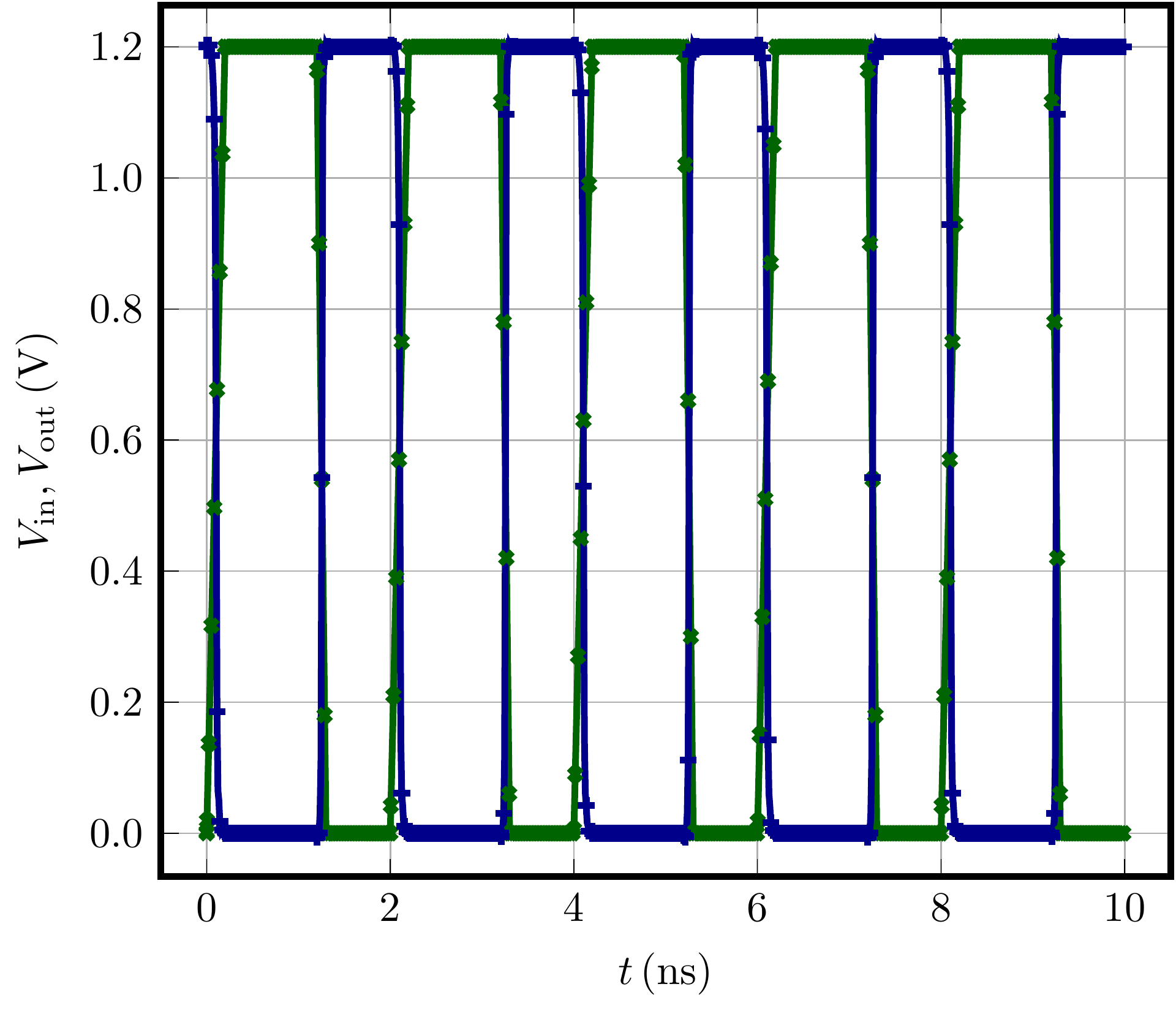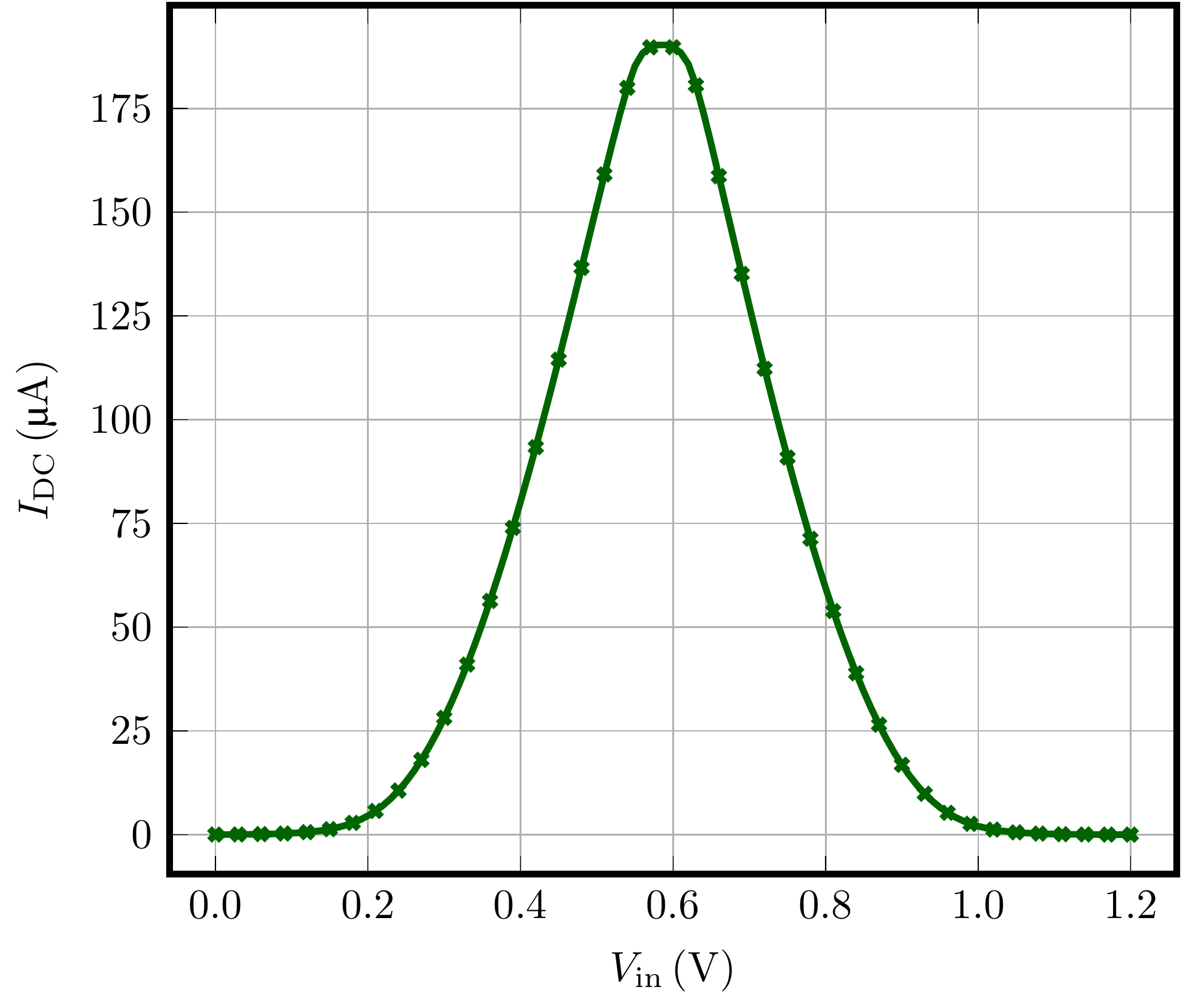# Examples

In the following, some example simulation that rely on Verilog-A defined compact models are shown. The Verilog-A models are compiled with OpenVAF and Ngspice is used for simulation. For this purpose, please install Ngspice as is explained on the Ngspice website.

Note: Support for OSDI/OpenVAF is currently only available in the pre-master branch of Ngspice until Ngspice-39 releases at the beginning of 2023.

You can obtain the sourecode for this version by running the following commands:

``````git clone git://git.code.sf.net/p/ngspice/ngspice
cd ngspice
git checkout pre-master
``````

## Example 1: HICUM/L2 Model

This example demonstrates how to simulate the HICUM/L2 model using OpenVAF.
First, create a folder that will be used for the simulation.

In this folder, place the following three files:

• The model parameter file model.l that specifies the model parameters and can be downloaded here.
``````OSDI Example

VB B  0 DC 0.1 AC 1 SIN (0.5 0.4 1M)
VC C  0 DC 1

.model npn_full_sh hicuml2va
.include model.l

N1 C B 0 0 npn_full_sh

.control
pre_osdi hicumL2V3p0p0.osdi

dc VC 0 2 0.01 VB 0.65 0.9 0.05 ;
plot -i(VC)
.endc

.end
``````

This netlist specifies a bipolar transistor that uses the model hicuml2va which is defined in the Verilog-A source file. The Verilog-A model is made available to Ngspice using the pre_osdi command.

For generating the osdi file you must compile the Verilog-A code by running

``````openvaf hicumL2V3p0p0.va
``````

in the simulation folder. This will generate the `hicumL2V3p0p0.osdi` file. Now you can run the circuit simulation using

``````ngspice netlist_osdi.sp
``````

and the output characteristics of the bipolar transistor are plotted:## Example 2: PSP MOS Inverter

This example shows the simulation of a MOS inverter using the PSP model. First, create a folder psp_inverter that will be used for the circuit simulation.

In this folder, place the following files:

• the netlist shown below, together with the example modelcards. These files can be download here.
• the PSP compact model Verilog-A source files can be downloaded here.

The tree structure of your folder should then look like this:

``````├── psp_inverter
│   ├── PSP103.8.0_vacode
│   │   ├── **/*
│   ├── Modelcards
│   │   ├── psp103_nmos.mod
│   │   ├── psp103_pmos.mod
│   ├── psp_inverter.sp
``````

This is the content of psp_inverter.sp:

``````* PSP models
* simple inverter

.param Vcc = 1.2
.csparam vcc='Vcc'

* Path to the models
.include Modelcards/psp103_nmos.mod
.include Modelcards/psp103_pmos.mod

* the voltage sources:
Vdd vdd gnd DC 'Vcc'
V1 in gnd pulse(0 'Vcc' 0p 200p 100p 1n 2n)
Vmeas vss 0 0

Xnot1 in vdd vss out not1
*Rout out 0 1k

.subckt not1 a vdd vss z
*m01   z a     vdd     vdd pch  l=0.1u  w=1u  as=0.26235  ad=0.26235  ps=2.51   pd=2.51
nmp1  z a     vdd     vdd pch
+l=0.1u
+w=1u
+sa=0.0e+00
+sb=0.0e+00
+absource=1.0e-12
+lssource=1.0e-06
+lgsource=1.0e-06
+abdrain=1.0e-12
+lsdrain=1.0e-06
+lgdrain=1.0e-06
+mult=1.0e+00

*m02   z a     vss     vss nch  l=0.1u  w=0.5u as=0.131175 ad=0.131175 ps=1.52   pd=1.52
nmn1  z a     vss     vss nch
+l=0.1u
+w=1u
+sa=0.0e+00
+sb=0.0e+00
+absource=1.0e-12
+lssource=1.0e-06
+lgsource=1.0e-06
+abdrain=1.0e-12
+lsdrain=1.0e-06
+lgdrain=1.0e-06
+mult=1.0e+00
c3  a     vss   0.384f
c2  z     vss   0.576f
.ends

* simulation command:
.tran 10ps 10ns
.dc V1 0 'vcc' 'vcc/100'

.control
pre_osdi PSP103.8.0_vacode/vacode/psp103.osdi
run
*set nolegend
plot in out
plot dc1.out
plot dc1.i(Vmeas)
rusage
.endc

.end

``````

Next, cd into the folder and run the following commands

``````cd psp_inverter/PSP103.8.0_vacode/vacode/
openvaf psp103.va
``````

This will compile the Verilog-A source file to psp103.osdi file for usage in Ngspice. Now, in the simulation folder, run

``````cd psp_inverter
ngspice psp_inverter.sp
``````

to calculate and plot the inverter characteristics:## Example 3: ISCAS85 Benchmark Circuit

In this example the massive ISCAS85 benchmark circuit that is often used for benchmarking circuit simulators is simulated. First, create a folder iscas85_benchmark_circuit that will be used for the circuit simulation. In this folder place the following files:

• the netlist together with the example modelcards that can be download here.
• the PSP compact model Verilog-A source files can be downloaded here.
``````├── iscas85_benchmark_circuit
│   ├── PSP103.8.0_vacode
│   │   ├── **/*
│   ├── Modelcards
│   │   ├── psp103_nmos.mod
│   │   ├── psp103_pmos.mod
│   ├── iscas85_benchmark_circuit.sp
``````

Next, cd into the folder and run the following commands

``````cd iscas85_benchmark_circuit/PSP103.8.0_vacode/vacode/
openvaf psp103.va
``````

This will compile the Verilog-A source file to psp103.osdi file for usage in Ngspice. Now, in the simulation folder, run

``````cd iscas85_benchmark_circuit
ngspice iscas85_benchmark_circuit.sp
``````

to see the results. Note that this is a very large circuit whose simulation may take a long time.